Contemporary Mathematics

# Chapter Test

### Chapter Test

1 .
Expand the Hindu-Arabic numeral 5,789.
2 .
Evaluate the expression $4 \times {7^3} + 5 \times {2^5}$.
3 .
Rewrite $6 \times {10^5} + 0 \times {10^4} + 8 \times {10^3} + 0 \times {10^2} + 1 \times {10^1} + 9 \times {10^0}$ in Hindu-Arabic form.
4 .
Convert the Babylonian numeral to a Hindu-Arabic numeral.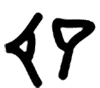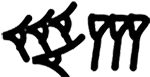5 .
Convert the Mayan number to a Hindu Arabic numeral.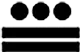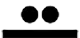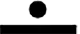6 .
What base system did the Babylonians use?
7 .
Which system—Roman, Babylonian, Mayan—used place values?
8 .
Convert the Roman numeral MDXLVII to a Hindu-Arabic numeral.
9 .
How many symbols are needed for a base 9 system?
10 .
For a system in a base larger than 10, what symbols are used as digits representing more than 10?
11 .
Convert 1328 to a base 10 number.
12 .
List the numbers in base 4 up to 1004.
13 .
Convert 74 to a base 12 number.
14 .
Create the addition table for base 4.
15 .
Calculate ${314_6} + {453_6}$.
16 .
Calculate 4B112 − 2A612.
17 .
When calculating ${23_6} + {53_6}$, a student obtains 766 . How do you know an error was made?
18 .
Create the multiplication table for base 4.
19 .
Calculate ${323_4} \times {132_4}$.
20 .
Calculate ${21_4} \div {3_4}$.
Order a print copy

As an Amazon Associate we earn from qualifying purchases.